# Electronics and Communication Engineering - Exam Questions Papers

41.

The switching expression corresponding to :
f(A, B, C, D)= ∑(0, 3, 4, 7, 8) +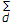(10, 11, 12, 14, 15) is:

 A. C D + B C B. C ⊙ D C. C ⊕ D D. CD

Explanation: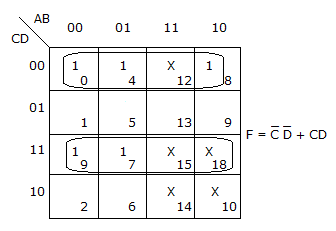42.

For each of the positive edge-triggered J-K flip flop used in the following figure, the propagation delay is ΔT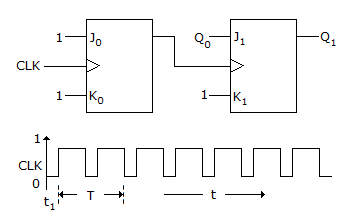Which of the following waveforms correctly represents the output at Q1?

 A.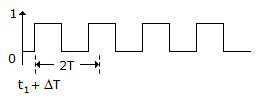B.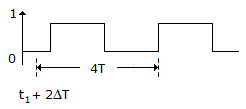C.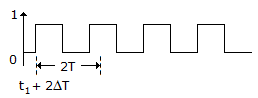D.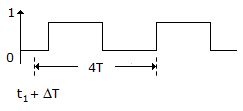Explanation:

Two JK flip flop. Thus propagation delay will be 2ΔT

Frequency is getting reduced by a factor e.

43.

Given the function F(A, B, C, D) =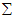m(0, 1, 2, 3, 4, 6, 8, 9, 10, 11, 12). The minimized form of the function can be given by :

 A. A + D B + C B B. (B + C)(A + B + C) C. (B + D)(A + B + C) D. C B + A D + B

Explanation:

Given S(A, B, C, D) =m(0, 1, 2, 3, 4, 6, 8, 9, 10, 11, 12)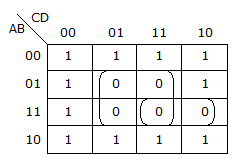S = (B + D)(A + B + C) .

44.

For the network shown, determine z1 , z0 and Av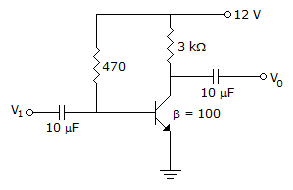A. zi = 1.067 Ω; z0 = 3 kΩ; Av = 280 B. zi = 1.067 Ω; z0 = 3 kΩ; Av = - 280.37 C. zi = 937 Ω; z0 = 3 Ω; Av = 281 D. zi = 937 Ω; z0 = 33 kΩ; Av = - 280.37

Explanation: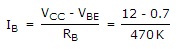= 24.04 μA

IE = (1 + β)IB = 2.428 mA;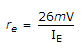= 10.7 Ω

(i) zi = RB||Bre =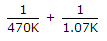= 0.937 K = 1.067 K

(ii) Z0 = RC = 3 kΩ

(iii)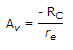= -280.37

Zi = 1.067 KΩ;

Z0 = 3 KΩ;

Av = -280.37 .

45.

A germanium diode has a reverse saturation current of 30 μA at 125°C and forward current of 1 mA at a forward potential of 0.8 V and the reverse potential is 0.6 V What are its dynamic forward and reverse resistance at this temperature.

 A. 10.7 Ω, 70 kΩ B. 34 Ω, 20 kΩ C. 80 Ω, 20 kΩ D. 800 Ω, 40 kΩ

Explanation:

We have,

I = I0•(eV/nVT -1)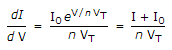For forward voltage greater than few teeth's of volts, I >> I0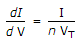Forward dynamic resistance,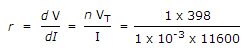= 34.3 Ω

Reverse dynamic resistance =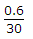= 20 kΩ.

#### Current Affairs 2022

Interview Questions and Answers